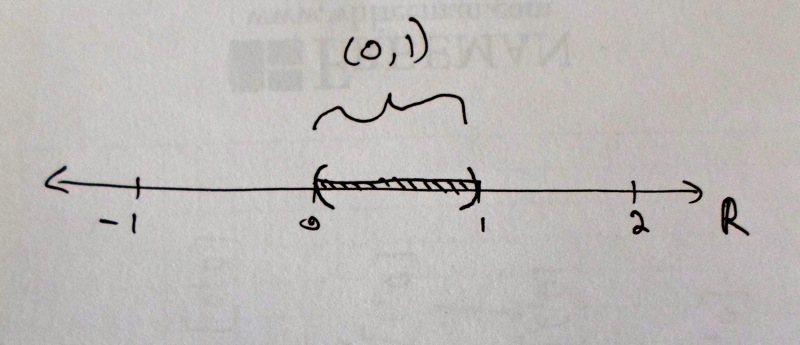# Second

Claim: breathed infinity is larger than digital infinity; or, $$|(0,1)| > |\mathbb{N}|$$.

Breathed infinity is the infinity of instants in a single breath. Every moment of lived consciousness that passes from the beginning of an inhale to the end of an exhale. It is an uninterrupted flow of points, a brief segment of a continuum. Let's denote this set by $$\mathscr{B}$$.

To make $$\mathscr{B}$$ more mathematically precise, we imagine it as a piece of the continuous real line that represents time. Or imagine looking at an analog stopwatch run as you breathe in and then breathe out; the movement of the watch hand carves out a line segment, capturing every instant along the way.

For the sake of simplicity, assume your breath takes one second. Ignore $$t=0$$ and $$t=1$$, but take every instant between $$0$$ and $$1$$. This set, thought of as a subset of the real line, is written $$(0,1)$$.So we will assume $$\mathscr{B}$$ is the same size as $$(0,1)$$.

And in the first module, we showed that digital infinity is the same size as $$\mathbb{N}$$.

Thus we will justify the claim that breathed infinity is larger than digital infinity, by proving the mathematical statement $$|(0,1)| > |\mathbb{N}|$$.

Top